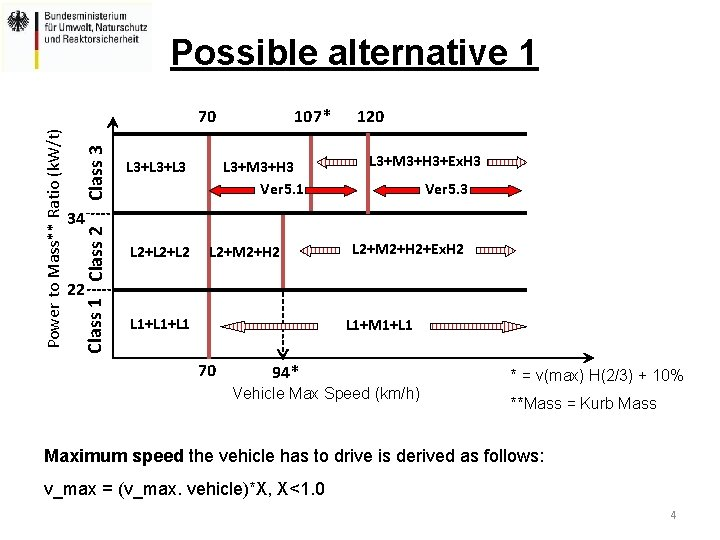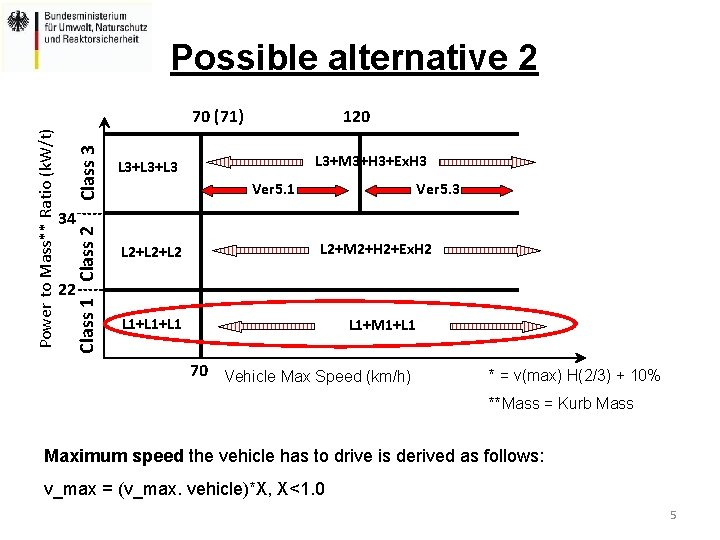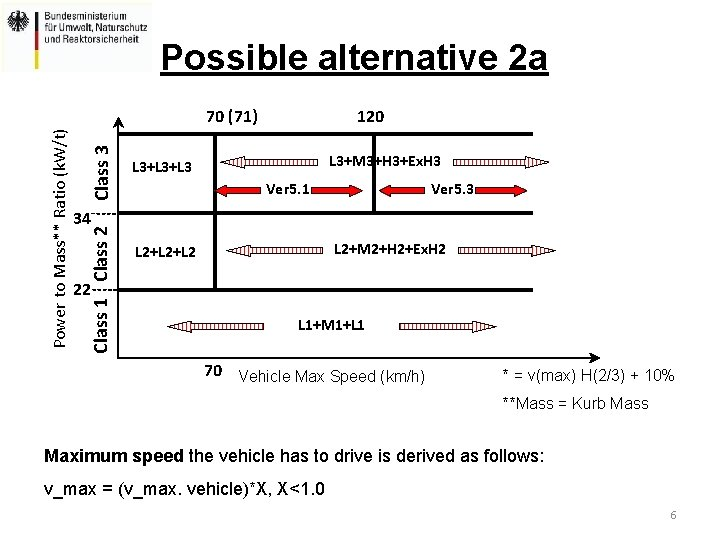# WLTPDHC16 04 Cycle allocation scheme Dr Oliver Eberhardt

• Slides: 7WLTP-DHC-16 -04 Cycle allocation scheme Dr. Oliver Eberhardt For the Federal Ministry for the Environment, Nature Conservation and Nuclear Safety Division IG I 6 "Air Pollution Control Technology in in the Field of Transport and Fuels, Biofuels"State of play Current cycle allocation scheme may lead to some “breaking points” with regard to the applied cycle phases to the different classes (2+3). This might mislead to special design of vehicles to reduce CO 2 emissions and fuel consumption applying different WLTC cycle phases. 2Analysis • Some contracting parties (e. g. IND and JPN) as well as OICA emphasized the need for a certain threshold between the maximum speed of the vehicle and the maximum cycle speed to avoid to too high percentage of wide open throttle operation. • The “vehicle maximum speed boundary” (red lines in graph) would this way be defined as follows for the different vehicle classes: – v_max (vehicle, boundary) = v_max (cycle phase) * [1, 10] -> proposal was agreed in principle, however with reservation with regard to the height of the margin as the applied cap leads to lower CO 2 emissions. • Application of a “safety margin” could lead to: – the “vehicle maximum speed boundaries” would be: v_max (vehicle) = v_max (cycle phase) * [1, 10] – the maximum cycle speed for the vehicle within those boundaries would be capped at: v_max (cycle phase) = v_max (vehicle)/(1 + X) (X < 0, 1) -> in order to reasonably restrict the possible CO 2 “benefit” X must be < 0. 1! – Example for X = 7% and v_max (vehicle) = 110 km/h: v_max (cycle phase) = 110 km/h / 1, 07 = 102, 8 km/h 3Possible alternative 1 Class 3 L 3+L 3 107* L 3+M 3+H 3 Ver 5. 1 120 L 3+M 3+H 3+Ex. H 3 Ver 5. 3 34 Class 1 Class 2 Power to Mass** Ratio (k. W/t) 70 L 2+L 2 L 2+M 2+H 2+Ex. H 2 22 L 1+L 1 L 1+M 1+L 1 70 94* Vehicle Max Speed (km/h) * = v(max) H(2/3) + 10% **Mass = Kurb Mass Maximum speed the vehicle has to drive is derived as follows: v_max = (v_max. vehicle)*X, X<1. 0 4Possible alternative 2 Class 3 120 L 3+M 3+H 3+Ex. H 3 L 3+L 3 Ver 5. 1 Ver 5. 3 34 Class 1 Class 2 Power to Mass** Ratio (k. W/t) 70 (71) L 2+L 2 L 2+M 2+H 2+Ex. H 2 22 L 1+L 1 L 1+M 1+L 1 70 Vehicle Max Speed (km/h) * = v(max) H(2/3) + 10% **Mass = Kurb Mass Maximum speed the vehicle has to drive is derived as follows: v_max = (v_max. vehicle)*X, X<1. 0 5Possible alternative 2 a Class 3 120 L 3+M 3+H 3+Ex. H 3 L 3+L 3 Ver 5. 1 Ver 5. 3 34 Class 1 Class 2 Power to Mass** Ratio (k. W/t) 70 (71) L 2+M 2+H 2+Ex. H 2 L 2+L 2 22 L 1+M 1+L 1 70 Vehicle Max Speed (km/h) * = v(max) H(2/3) + 10% **Mass = Kurb Mass Maximum speed the vehicle has to drive is derived as follows: v_max = (v_max. vehicle)*X, X<1. 0 6How to possibly go further on? • Early decision on cycle allocation necessary not to delay WLTP GTR decision! • Quickly evaluate possible CO 2 impact of different approaches for decision. • Chosen approach shall not provide misleading vehicle design incentives or CO 2 benefits for manufacturers.